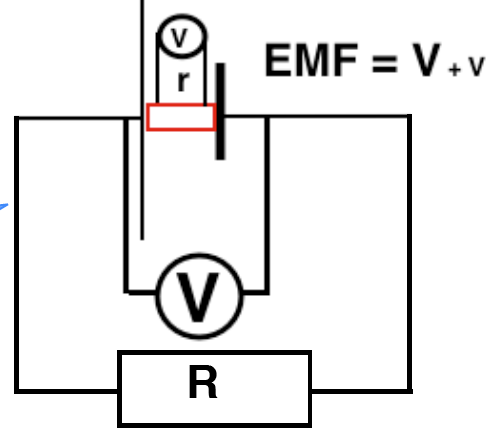EMF and Internal resistance

Internal resistance

Inside a dry cell there is a mix of gungy chemicals which convert chemical energy to electrical energy. The electric current that is produced still has to flow around all the circuit, including through the cell itself. These chemicals have a significant resistance called the “internal resistance”. Electrical generators and transformers also have an internal resistance due to the long coils of wire. The internal resistance of a car battery however is very small.

The current requires power (a PD) to push through this internal resistance as well as through the external  resistance.

EMF

If r is the internal resistance, then remember that the same current I flows round the whole circuit, so:

I = V/R = EMF/ R + rThe EMF is the total of the external PD (voltage) and the internal PD. It is the total energy supplied to each coulomb of charge flowing around the circuit including that needed to push through the supply itself.

We can measure this directly with a voltmeter (see below), but only if we assume that the voltmeter requires no current to function. (In fact modern digital meters require only a minute current to function so this method is very accurate for most purposes.)